# Distance Displacement Speed and Velocity Questions for Consideration

• Slides: 20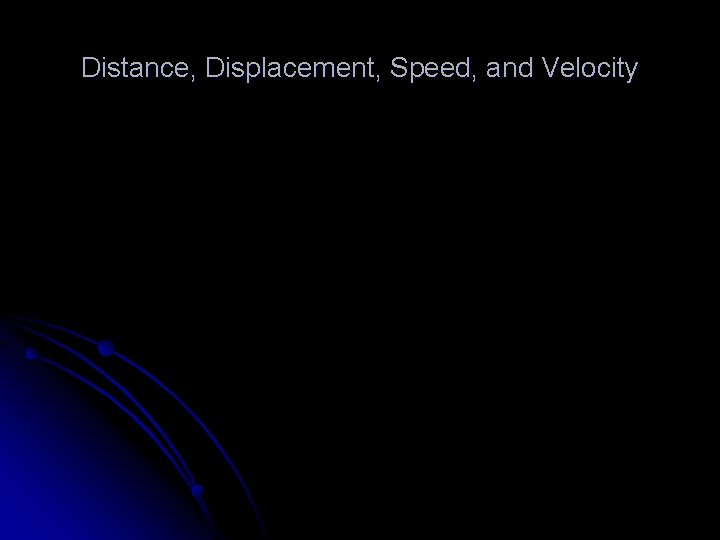Distance, Displacement, Speed, and VelocityQuestions for Consideration What is frame of reference? l What is distance? l How is displacement different from distance? l What is speed? l What is velocity? l What are scalar and vector quantities? l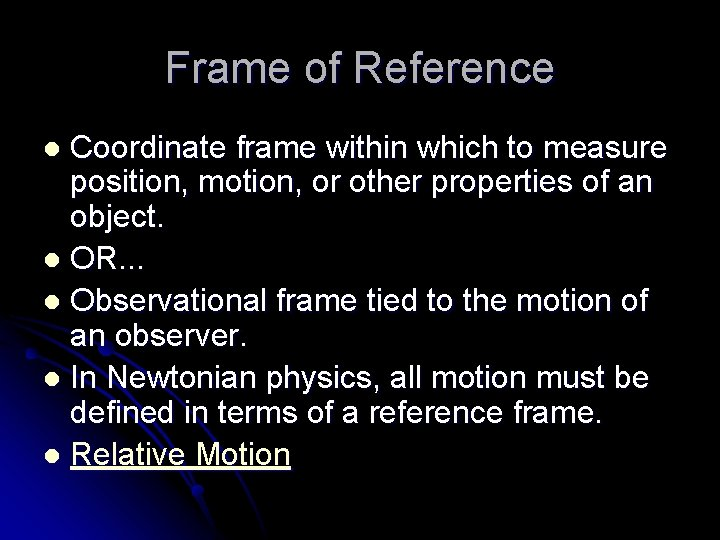Frame of Reference Coordinate frame within which to measure position, motion, or other properties of an object. l OR. . . l Observational frame tied to the motion of an observer. l In Newtonian physics, all motion must be defined in terms of a reference frame. l Relative Motion lReference Frame l Is the speed of the ball different relative to the pitcher, the truck driver, and the jet pilot? Why or why not?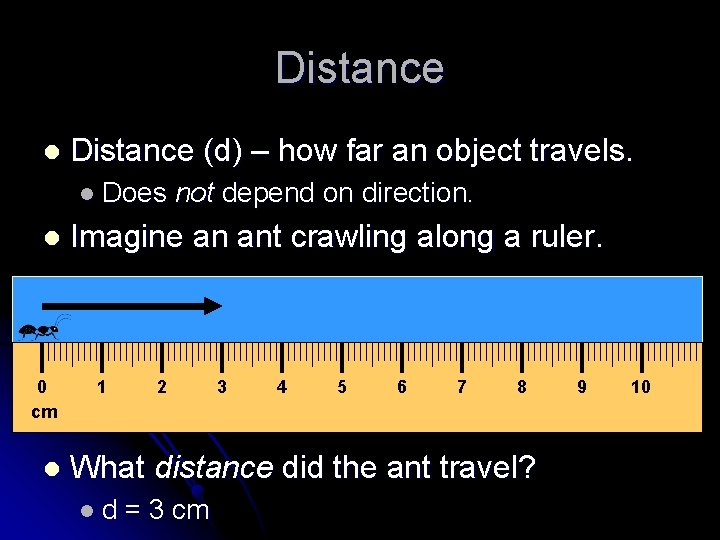Distance l Distance (d) – how far an object travels. l Does l 0 cm l not depend on direction. Imagine an ant crawling along a ruler. 1 2 3 4 5 6 7 8 What distance did the ant travel? ld = 3 cm 9 10Distance does not depend on direction. l Here’s our intrepid ant explorer again. l 0 cm l 1 3 4 5 6 7 8 9 Now what distance did the ant travel? ld l 2 = 3 cm Does his direction change the answer? 10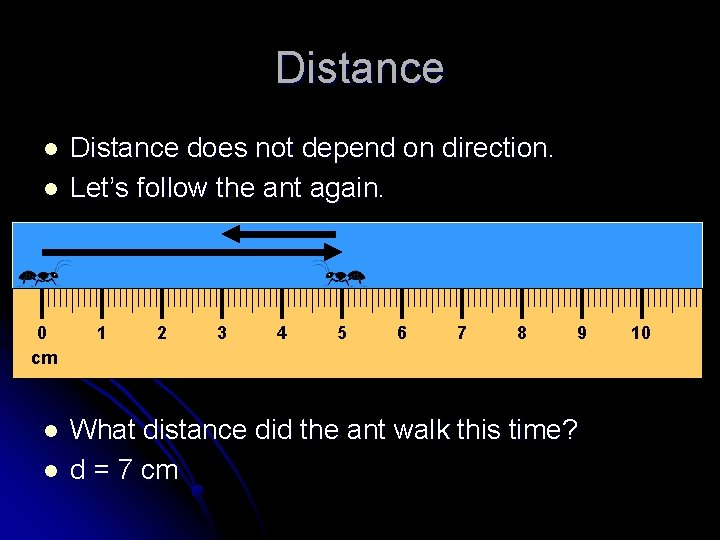Distance l l 0 cm l l Distance does not depend on direction. Let’s follow the ant again. 1 2 3 4 5 6 7 8 9 What distance did the ant walk this time? d = 7 cm 10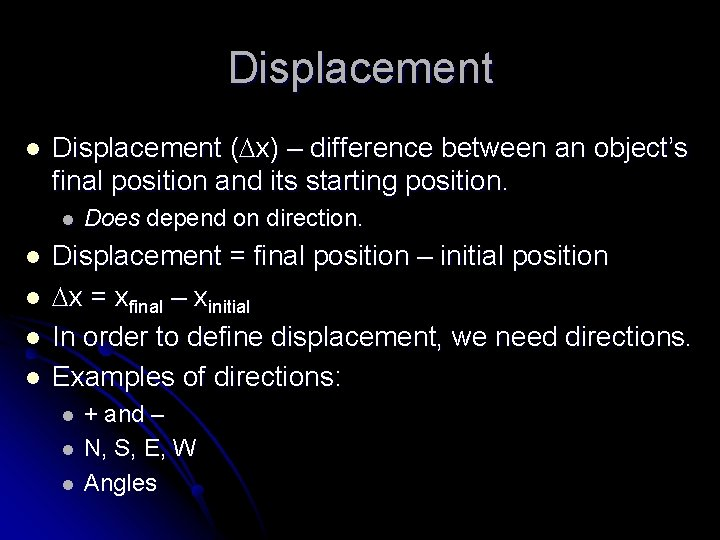Displacement l Displacement ( x) – difference between an object’s final position and its starting position. l l l Does depend on direction. Displacement = final position – initial position x = xfinal – xinitial In order to define displacement, we need directions. Examples of directions: l l l + and – N, S, E, W AnglesDisplacement vs. Distance l Example of distance: l The l Example of displacement: l The l ant walked 3 cm EAST. An object’s distance traveled and its displacement aren’t always the same!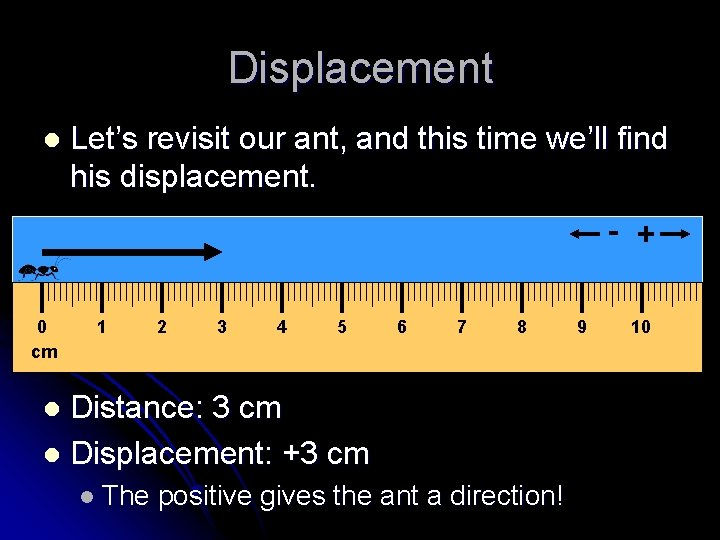Displacement l Let’s revisit our ant, and this time we’ll find his displacement. - + 0 cm 1 2 3 4 5 6 7 8 Distance: 3 cm l Displacement: +3 cm l l The positive gives the ant a direction! 9 10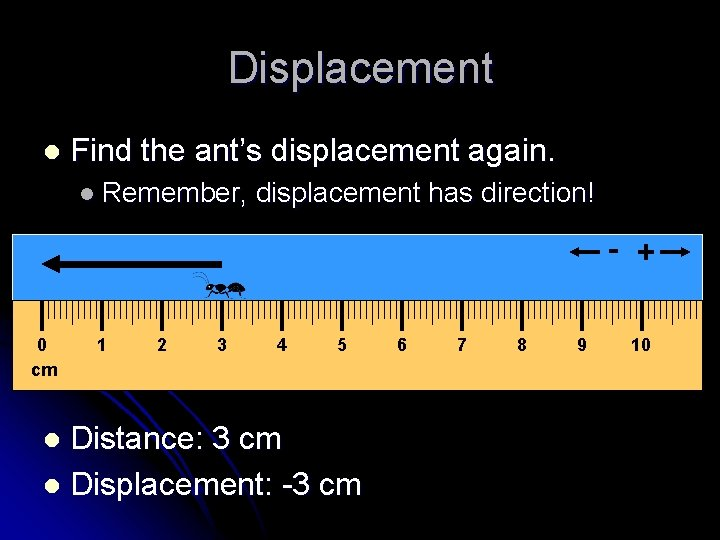Displacement l Find the ant’s displacement again. l Remember, displacement has direction! - + 0 cm 1 2 3 4 5 Distance: 3 cm l Displacement: -3 cm l 6 7 8 9 10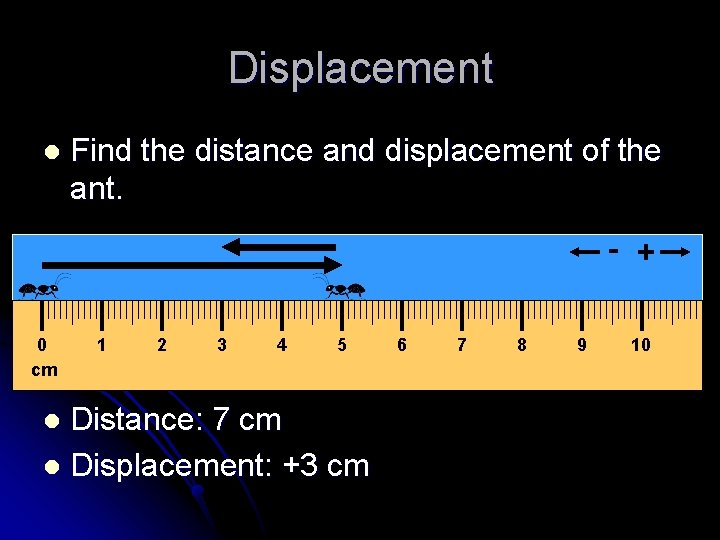Displacement l Find the distance and displacement of the ant. - + 0 cm 1 2 3 4 5 Distance: 7 cm l Displacement: +3 cm l 6 7 8 9 10Displacement vs. Distance l An athlete runs around a track that is 100 meters long three times, then stops. l What is the athlete’s distance and displacement? l l l Distance = 300 m Displacement = 0 m Why?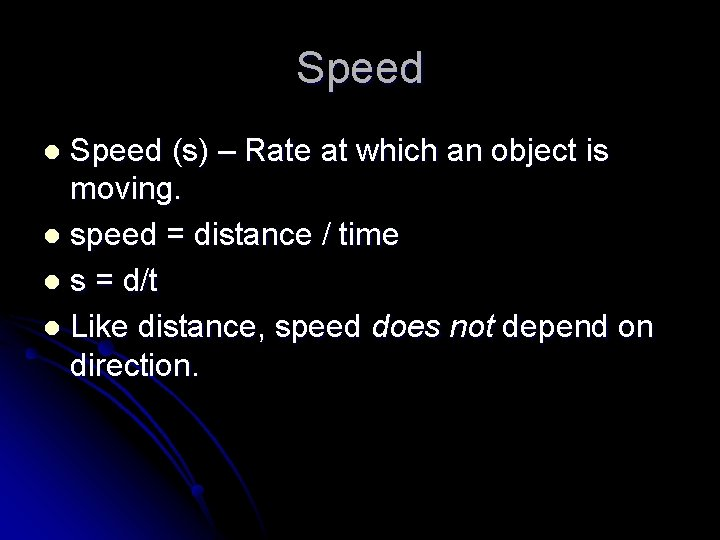Speed (s) – Rate at which an object is moving. l speed = distance / time l s = d/t l Like distance, speed does not depend on direction. lSpeed l A car drives 100 meters in 5 seconds. 1 s 2 3 4 5 100 m l What is the car’s average speed? ls = d/t l s = (100 m) / (5 s) = 20 m/s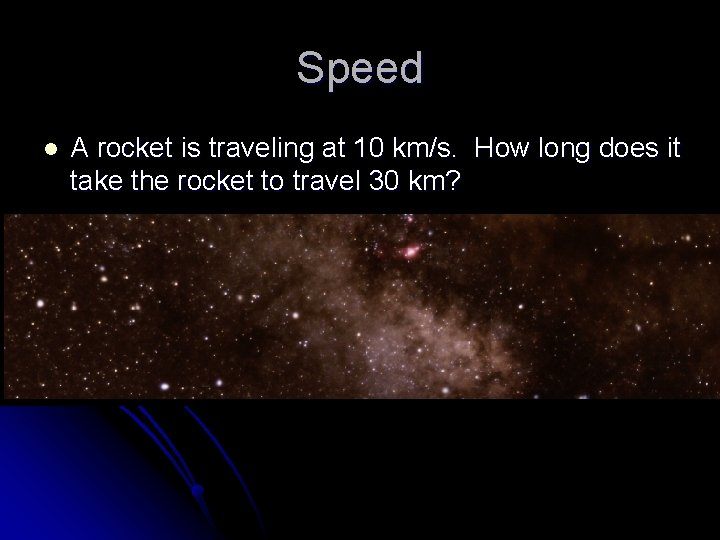Speed l A rocket is traveling at 10 km/s. How long does it take the rocket to travel 30 km?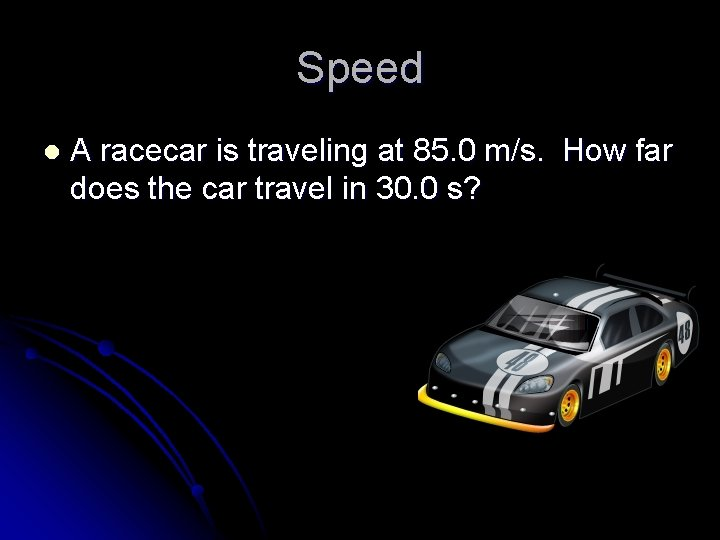Speed l A racecar is traveling at 85. 0 m/s. How far does the car travel in 30. 0 s?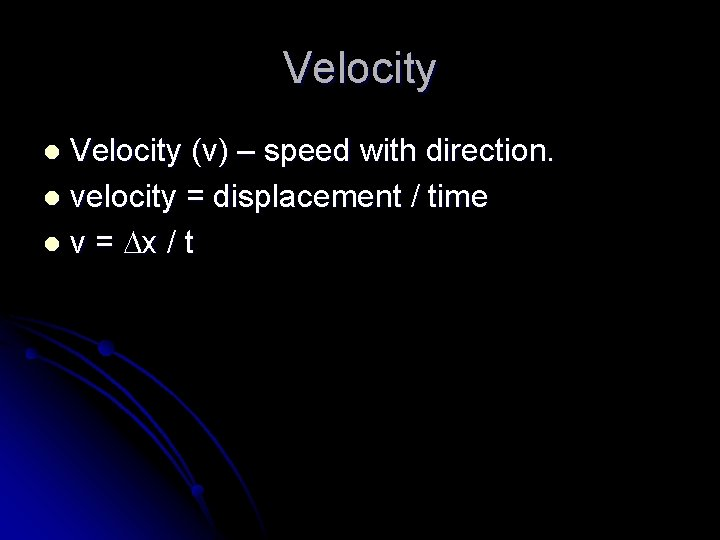Velocity (v) – speed with direction. l velocity = displacement / time l v = x / t lPulling It All Together l Back to our ant explorer! - + 1 s 2 3 4 5 0 cm 1 2 l Distance 3 4 5 6 7 8 9 10 traveled: 7 cm l Displacement: +3 cm l Average speed: (7 cm) / (5 s) = 1. 4 cm/s l Average velocity: (+3 cm) / (5 s) = +0. 6 cm/sScalar and Vector Quantities l Scalar Quantity – has magnitude but not direction. l Distance l and speed are scalar quantities. Vector Quantity – has magnitude and direction. l Displacement quantities. and velocity are vector